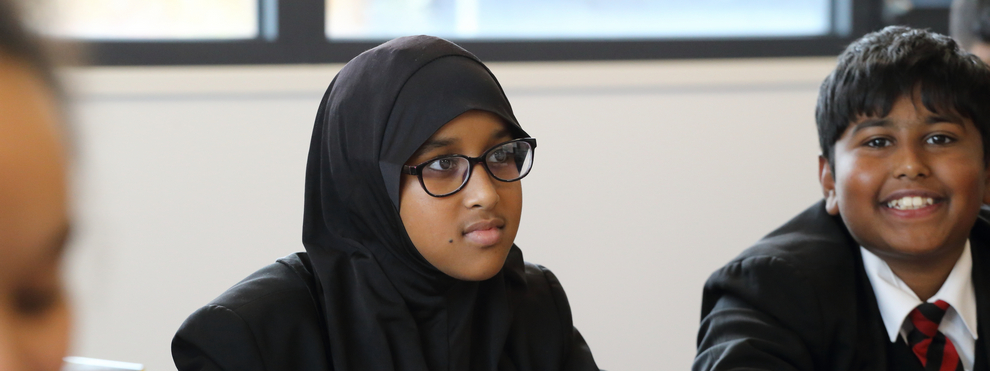# Mathematics### Mathematics

Students should become fluent in the fundamentals of mathematics, being able to perform increasingly complex mathematical calculations with precision and mastery. They should understand mathematical terminology.

Students should also learn how to reason mathematically and to use mathematical knowledge to solve routine and non-routine problems. They will learn to develop an argument and provide reasonable justification or proof.

Overview

 Year Term Topic(s) Year 7 Autumn Unit 1  : Numbers and CalculationsWhole numbers and decimalsWhole number calculationsFactors and multiplesFractions, decimals and percentages Spring Unit 2: Algebra and GraphsExpressions and formulaeGraphsEquations Summer Unit 3: Measure, Perimeter and AreaAngles and 2D shapes Transformations and symmetry Unit 4: Statistics and Probability Year 8 Autumn Unit 1: Numbers and CalculationsWhole numbers and decimalsWhole number calculationsFactors and multiplesFractions, decimals and percentages Spring Unit 2: Algebra and GraphsExpressions and formulaeGraphsEquations Summer Unit 3: Measure, Perimeter and AreaAngles and 2D shapesTransformations and symmetry Unit 4: Statistics and Probability Year 9 Autumn Unit 1: Number  Powers, decimals, HCF and LCM, positive and negative, roots, rounding, reciprocals, standard form, indices and surds Spring Unit 2:  AlgebraExpressions, substituting into simple formulae, expanding and factorising, equations, sequences and inequalities, simple proof, rearranging and solving equations Summer Unit 3:  StatisticsDrawing and interpreting graphs, tables and charts, averages and range, collecting data, representing data Year 10 Autumn Geometry 2 (Higher)Measure and Statistics 2 (Foundation) Spring Unit 8: Geometry and Measures (Higher),Unit 9: Algebra 3 (Foundation)Unit 10 Geometry and measures 3Transformations, constructions loci and bearings Summer Unit 10: Probability (Higher)Unit 13: (Foundation)Unit 8: Geometry and Measures (Higher)Unit 10 Geometry and measures 3 (Foundation)Ratio and proportionsUnit 9: Algebra 3 (Foundation) Year 11 Autumn Statistics 2 (Higher)Geometry and Measures 4 (Foundation)Unit 15: Geometry and Measures 4 (Foundation)Collecting data, cumulative frequency, quadratics, plans, elevations and nets Spring Circles, cylinders, cones and squaresGeometry and circle theoremsCircle geometryIndices and standard formAlgebra Summer Geometry vectorsBasic geometryGeometric proofReciprocal and exponential graphsGradientSingularity and congruence in 2D

GCSE courses:

Mathematics Edexcel (1MA1)

Further Mathematics AQA (8365)